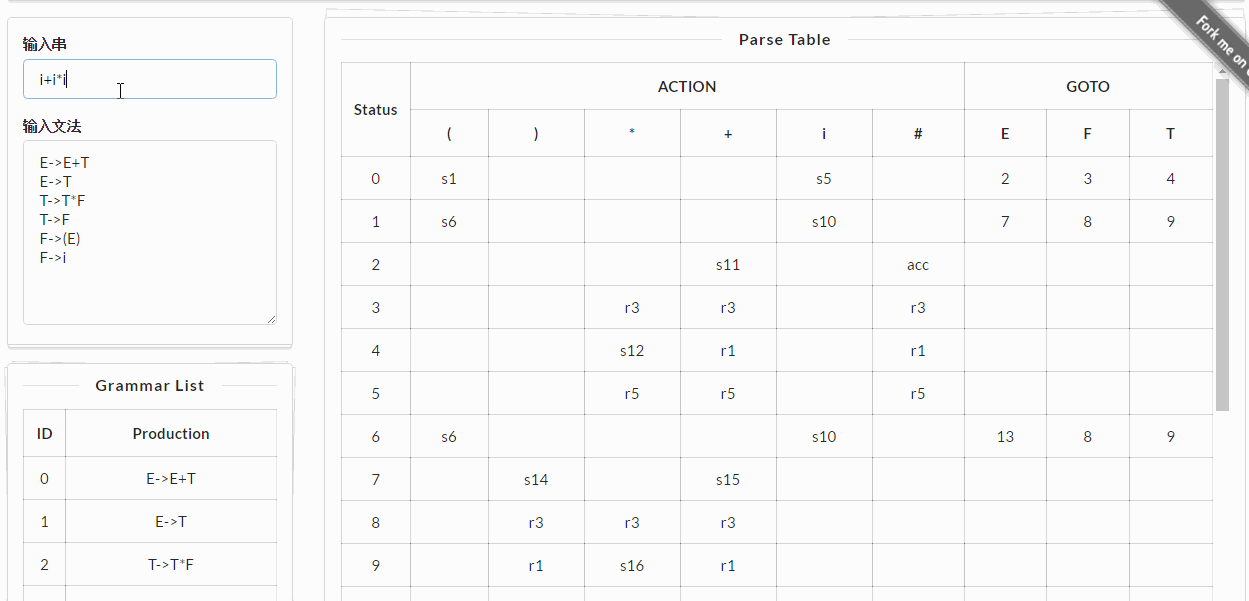## 产生式Prod类

class Prod { // 这里是存放形如X->abc的形式，不存在多个候选式
private:
char noTerminal; // 产生式左部非终结符名字
string right; // 产生式右部
friend bool operator == (const Prod &a, const Prod &b) {
return a.noTerminal == b.noTerminal && a.right == b.right;
}
friend bool operator == (const Prod &a, char c) {
return a.noTerminal == c;
}
public:
Prod(const char &noTerminal, const string& right, const set<char>& additionalVt):
};


## 项目集Item类

class Item { // 项目集
private:
vector<Prod> prods; // 项目集产生式
static set<char> Vn; // 非终结符
static set<char> Vt; // 终结符
static set<char> Symbol; // 所有符号
friend bool operator == (const Item &a, const Item &b) {
if(a.prods.size() != b.prods.size()) return false;
else {
for(const auto& p: a.prods) {// 选择a的每个产生式
auto it = find(b.prods.begin(), b.prods.end(), p);
if(it == b.prods.end())  // 找不到
return false;
else  {// 找到的话判断附加终结符是否都相等
return false;
}
}
return true;
}
}
public:
};


## LR类

class LR {
private:
Item G; // 文法G
enum actionStat{
ACCEPT=0,
SHIFT,
REDUCE,
};

vector<Item> C; // 项目集规范族
map<pair<int, char>, int> GOTO; // goto数组，项目集<int, int>=char
map<pair<int, char>, pair<actionStat, int> > ACTION; // Action数组，Action[(i, a)]=(s|r)j
map<char, set<char> > FIRST; // first集
set<char> first(const string &s); // 求first集
vector<char> inStr; // 输入串/栈
vector<int> status; // 状态栈
vector<char> parse; // 分析栈
Item closure(Item I); // 求该项目的闭包
Item Goto(const Item& I, char X); // 求I经过X到达的项目集
void items(); // 求项目集状态机DFA！!
public:
void add(const string &s); // 添加产生式
void parser(); // LR(1)分析
};


## PL0文法测试

A->B,
B->CEFH
B->H
B->CH
B->EH
B->FH
B->CFH
B->CEH
B->EFH
C->cY;
D->b=a
E->dX;
F->GB;
G->eb;
H->I
H->R
H->T
H->S
H->U
H->V
H->J
I->btL
J->fWg
K->LQL
K->hL
L->LOM
L->M
L->-M
L->+M
M->MPN
M->N
N->b
N->a
N->(L)
O->+
O->-
P->*
P->/
Q->=
Q->%
Q-><
Q->r
Q->>
Q->s
R->pKqH
S->mb
T->nKoH
U->i(X)
V->j(Z)
W->W;H
W->H
X->X,b
X->b
Y->Y,D
Y->D
Z->Z,L
Z->L## for auto & Bug

#include <iostream>
#include <vector>
using namespace std;

int main() {
vector<int> v={1, 2, 3};
for(const auto &i: v) {
printf("i=%d\n", i);
v.push_back(5);
printf("i=%d\n", i);
}
return 0;
}


i=1
i=0
i=0
i=0
i=3
i=3


i=0是哪来的。。。这个没找出原因，最后简单的用for(int i=0; ...)来替代处理了。

If the new size() is greater than capacity() then all iterators and references (including the past-the-end iterator) are invalidated. Otherwise only the past-the-end iterator is invalidated.